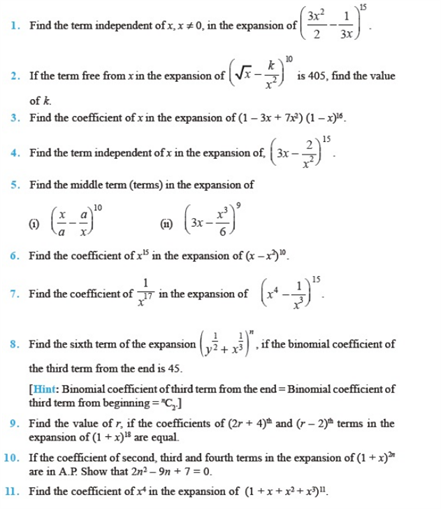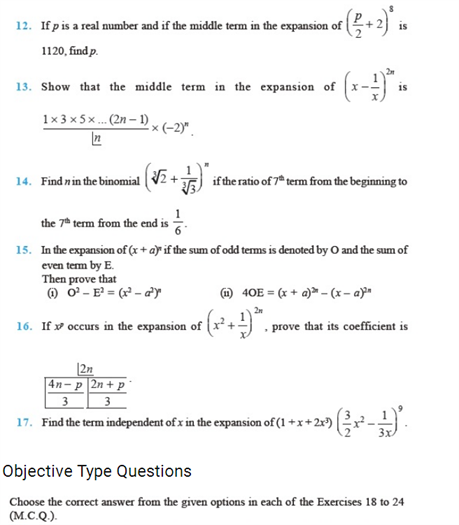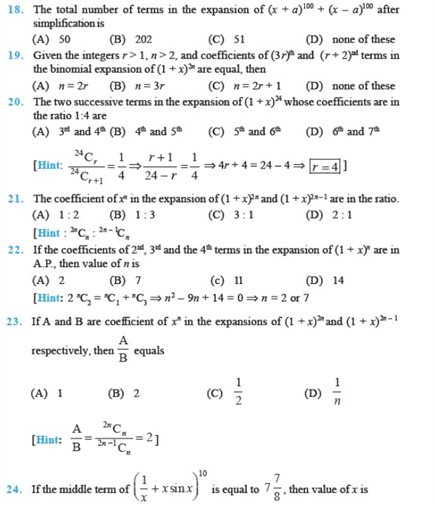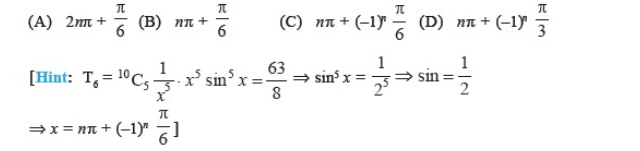# Chapter 8 - Binomial Theorem

Find the most important Class 11 Binomial chapter – 8 questions here. Chapter 7 of class 11 covers all types of Binomial theorem’s Problems. These important questions from class 11 will help you prepare for board exams of Class 12 too(CBSE) as you understand the concept of Binomial thoroughly.

You may also check the related topics like:

## Binomial Theorem Important Questions Class 11 Chapter 7

These questions given below are given to make you confident about the topic. You’ll be able to solve all types of class 11 chapter 7 questions if you practice these NCERT and other given questions Properly.

1. Expand (2a – 3b)4 by binomial theorem.

2. Using Binomial theorem, expand (a + 1/b)11.

3. Write the general term in the expansion of (a2 – b )6.

4. Find x, if T11 and T12 in the expansion of (2+ x)50 are equal.

5. The coefficients of three consecutive terms in the expansion of (1 + a)n are in the ratio 1:7:42. Find n.

### NCERT Questions on Binomial Theorem Class 11